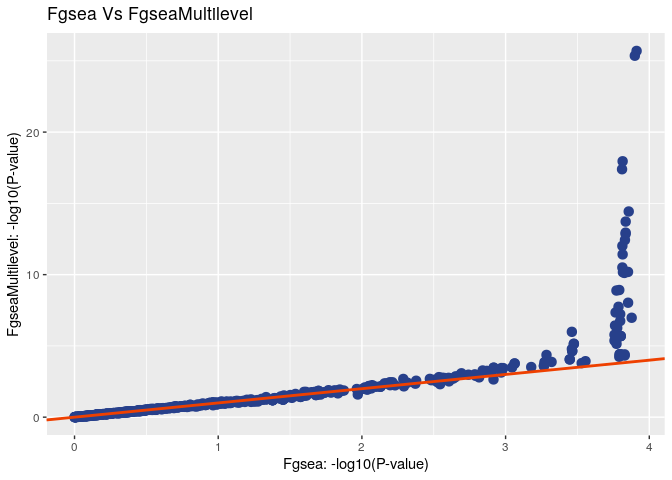# Using fgseaMultilevel function

#### 2018-11-14

fgseaMultilevel is a new function in fgsea package. The basis of this function is the adaptive multilevel splitting Monte Carlo approach. This approach allows us to exceed the results of simple sampling and calculate arbitrarily small P-value.

library(fgsea)
library(ggplot2)
library(BiocParallel)
register(SerialParam())

## Quick run

data(examplePathways)
data(exampleRanks)

Running fgseaMultilevel:

fgseaMultilevelRes <- fgseaMultilevel(pathways = examplePathways,
stats = exampleRanks,
minSize=15,
maxSize=500)

The resulting table contains enrichment scores and p-values:

head(fgseaMultilevelRes[order(pval), ])
##                                            pathway         pval
## 1:                              5990980_Cell_Cycle 5.741082e-27
## 2:                     5990979_Cell_Cycle,_Mitotic 7.539587e-26
## 3:                    5991851_Mitotic_Prometaphase 9.435196e-19
## 4: 5992217_Resolution_of_Sister_Chromatid_Cohesion 1.531671e-17
## 5:                                 5991454_M_Phase 1.522066e-14
## 6:         5991599_Separation_of_Sister_Chromatids 4.186360e-14
##            padj   log2err        ES      NES size
## 1: 3.364274e-24 1.3499257 0.5388497 2.699599  369
## 2: 2.209099e-23 1.3188888 0.5594755 2.774436  317
## 3: 1.843008e-16 1.1146645 0.7253270 2.971880   82
## 4: 2.243897e-15 1.0768682 0.7347987 2.940691   74
## 5: 1.783861e-12 0.9759947 0.5576247 2.576344  173
## 6: 4.088678e-12 0.9653278 0.6164600 2.682275  116
## 1: 66336,66977,12442,107995,66442,19361,...
## 2: 66336,66977,12442,107995,66442,12571,...
## 3: 66336,66977,12442,107995,66442,52276,...
## 4: 66336,66977,12442,107995,66442,52276,...
## 5: 66336,66977,12442,107995,66442,52276,...
## 6: 66336,66977,107995,66442,52276,67629,...

The output table is similar in structure to the table obtained using the fgsea function, except for the log2err column. This column corresponds to the standard deviation of the P-value logarithm.

## Function argument

• pathways - List of gene sets to check.
• stats - Named vector of gene-level stats. Names should be the same as in pathways
• sampleSize - The size of randomly generated gene sets, where each set has pathway size.
• minSize - Minimal size of a gene set to test. All pathways below the threshold are excluded.
• maxSize - Maximal size of a gene set to test. All pathways above the threshold are excluded.
• absEps - This parameter sets the boundary for calculating the p value.
• nproc - If not equal to zero sets BPPARAM to use nproc workers (default = 0).
• BPPARAM - Parallelization parameter used in bplapply.

Let $$q$$ be the initial set of genes of size $$k$$ for which we calculate the P-value. Then the general scheme of the algorithm is as follows:

• Random sets of $$k$$-size genes are generated in an amount equal to sampleSize. For each set of genes, an enrichment score (ES) is calculated. The median value of the ES for a given set of genes is then determined.
• The next step generates sets of genes with ES exceeding the median value obtained in the previous step. For newly generated samples, the median ES value is determined, and so on.

Let $$m_{k,j}$$ be the median value of ES for set of $$k$$-size genes obtained at the $$j$$ step. As a result, at some step, we obtain that the following relation will be satisfied for the initial set of genes: $m_{k,j} \leq ES(q) < m_{k,j+1}$ Then the P-value is in the range from $$2^{-j-1}$$ to $$2^{-j}$$. In addition, we can achieve even better accuracy by using not only the medians themselves, but also the intermediate data.

## Compare fgsea vs fgseaMultilevel

Compare fgsea with fgseaMultilevel on example data.

set.seed(42)

fgseaRes <- fgsea(pathways=examplePathways, stats=exampleRanks,
minSize=15, maxSize=500, nperm=10000)

fgseaMultilevelRes <- fgseaMultilevel(pathways=examplePathways,
stats=exampleRanks,
minSize=15, maxSize=500,
sampleSize=100)

logPvalsDf <- data.frame(fgseaData=-log10(fgseaRes$pval), fgseaMultilevelData=-log10(fgseaMultilevelRes$pval))

The following figure compares the P-value calculated using two approaches.

ggplot(logPvalsDf, aes(x=fgseaData, y=fgseaMultilevelData)) +
geom_point(color='royalblue4', size=3) +
xlab("Fgsea: -log10(P-value)") +
ylab("FgseaMultilevel: -log10(P-value)") +
geom_abline(size=1, color='orangered2') +
labs(title="Fgsea Vs FgseaMultilevel")It can be seen that up to a certain value, which is characterized by the parameter $$\dfrac{1}{nperm}$$, both algorithms give similar results. In this case, the accuracy of the P-value determination by the gsea algorithm is limited by the parameter $$\dfrac{1}{nperm}$$, while the fgseaMultilevel does not have these restrictions.